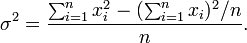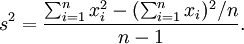﻿ Mathematical Functions
Nevron Open Vision Documentation
Mathematical Functions

Syntax Description Example
ABS(nmVar) Returns the absolute value of a number or measure.

ABS(-2.5)
Returns: 2.5

ABS(-2.5[mm])
Returns: 2.5 [millimeter]

AVG(nm1[,nm2,...nmN]) Returns the average of a set of numbers or measures. The arguments can be only number variants or only measure variants of the same dimension or arrays of such variants. Empty values are ignored from both the internal sum and count calculations.

AVG(10,15,20)
Returns: 15

AVG(ARRAY(10,15,20))
Returns: 15

AVG(ARRAY(10,15,EMPTY()),20,EMPTY())
Returns: 15

AVG(10[mm],15[in],20[mm])
Returns: 137 [millimeter]

CEILING(nmVar[, nMultiple]) Rounds a number or measure to the next (larger) instance of multiple. If multiple is not specified, the value rounds the next integer.

CEILING(1.7)
Returns: 2

CEILING(-1.7)
Returns: -1

CEILING(1.7, 0.25)
Returns: 1.75

CEILING(-1.7, 0.25)
Returns: -1.5

FLOOR(nmVar[, nMultiple]) Rounds a number or measure to the previous (smaller) instance of multiple. If multiple is not specified, the value rounds to the previous integer.

FLOOR(1.7)
Returns: 1

FLOOR(-1.7)
Returns: -2

FLOOR(1.7, 0.25)
Returns: 1.5

FLOOR(-1.7, 0.25)
Returns: -1.75

INT(nmVar[, nMultiple]) Rounds a number or measure to the adjacent instance of multiple that is closer to zero. If multiple is not specified, the value rounds to the previous integer. INT(1.7)
Returns: 1

INT(-1.7)
Returns: -1

INT(1.7, 0.25)
Returns: 1.5

INT(-1.7, 0.25)
Returns: -1.5
INTUP(nmVar[, nMultiple]) Rounds a number or measure to the adjacent instance of multiple that is more distant to zero. If multiple is not specified, the value rounds to the previous integer.

INTUP(1.7)
Returns: 2

INTUP(-1.7)
Returns: -2

INTUP(1.7, 0.25)
Returns: 1.75

INTUP(-1.7, 0.25)
Returns: -1.75

LN(nNumber) Returns the natural logarithm of a number. The number must be positive.

LN(10)
Returns: 2.3026

LOG10(nNumber) Returns the base 10 logarithm of a number. The number must be positive

LOG10(10)
Returns: 1

LOG(nNumber, nBase) Returns the logarithm of a number. The number and the base must be positive. LOG(256, 2)
Returns: 8
MAGNITUDE(nmA, nmB) Returns the magnitude of the vector whose rise is A and whose run is B. MAGNITUDE is calculated according to the following formula: SQRT(A^2 +  B^2)

MAGNITUDE(3, 4)

Returns: 5

MAX(var1[, var2, ..., varN]) Returns the largest variant from a set of variants. All variants in the set should be comparable to each other. Empty values are skipped from the calculation. If only empty values are provided the function returns empty.

MAX(1, 3, 2)
Returns: 3

MAX(ARRAY(1,3,2,EMPTY()))
Returns: 3

MAX(3[mm], 2[in], 1[m])
Returns: 1 [meter]

MEDIAN(n1[, n2, ..., nN]) Returns a value which splits a set of numbers. Half of the value are smaller than the median value and half are larger. Empty values are skipped from the calculation.

MEDIAN(1,3,2)
Returns: 2

MEDIAN(1,3,5,6)
Returns: 4

MIN(var1[, var2, ..., varN]) Returns the smallest variant from a set of variants. All variants in the set should be comparable to each other. Empty values are skipped from the calculation. If only empty values are provided the function returns empty.

MIN(1, 3, 2)
Returns: 1

MIN(ARRAY(1,3,2,EMPTY()))
Returns: 1

MIN(3[mm], 2[in], 1[m])
Returns: 3 [millimeter]

MOD(nmVar, nDivisor) Returns the remainder (modulus) resulting when a number or measure is divided by a divisor. The result has the same sign as the divisor.

MOD(5, 1.4)
Returns: 0.8

MOD(5, -1.4)
Returns -0.6

PERCENTILE(arr, fPercent) Returns a value which splits the set at arbitrary percent. {Percent count} of the values are smaller than this value and the other ones are larger. Percentile50 is equivalent to the Median formula. You can use the percentile to compute quartiles and deciles. 1 quartile is equivalent to Percentile25. 1 decile is equal to Percentile10.

PERCENTILE(ARRAY(25,10,20,30), 25)
Returns: 15

PERCENTILE(ARRAY(25,10,20,30), 75)
Returns: 27.5

POW(nmVar, fExponent) Returns a number or measure raised to the power of an exponent. POW(10, 2)
Returns: 100
ROUND(nmVar, nDigits).

Rounds a number or measure to the precision represented by digits.
If digits is greater than 0, number is rounded by digits to the right of the decimal.
If digits is 0, number is rounded to an integer.
If digits is less than 0, number is rounded by digits to the left of the decimal.

ROUND(123.654,2)
Returns: 123.65

ROUND(123.654,0)
Returns: 124

ROUND(123.654,-1)
Returns: 120

SIGN(nmVar[,fFuzz]) Returns a value that represents the sign of a number. Returns 1 if number is positive, 0 if number is zero, or -1 if number is negative. Fuzz (optional) helps avoid floating-point roundoff errors when a calculation is almost zero.

SIGN(-10)
Returns: -1.

SIGN(0)
Returns: 0

SQRT(nmVar) Returns the square root of a number or measure variant.

SQRT(4)
Returns: 2

SQRT(4 [mm^2])
Returns: 2 [millimeter]

STDDEV(arr, bEntirePopulation) Returns the standard deviation of the values in the set. It is computed as the positive square root of the variance.

STDDEV(ARRAY(4,7,13,16),true)
Returns: 4.74

STDDEV(ARRAY(4,7,13,16),false)
Returns: 5.48

SUM(nm1[, nm2, ..., nmN]) Returns the sum of a list of numbers or measures. Empty values are skipped from the calculation. If only empty values are provided the function returns empty. SUM(1,2,3)
Returns: 6

SUM(ARRAY(1,2,3,EMPTY()))
Returns: 6

SUM(ARRAY(EMPTY()))
Returns: empty
TRUNC(nmVar, fDigits) Returns a number truncated to number of digits.
If digits is greater than 0, number is truncated to number of digits to the right of the decimal.
If digits is 0, number is truncated to an integer.
If digits is less than 0, number is truncated to number of digits to the left of the decimal.

TRUNC(123.654,2)
Returns: 123.65.

TRUNC(123.654,0)
Returns: 123

TRUNC(123.654,-1)
Returns: 120

VARIANCE(arr, bEntirePopulation)

The variance is used to measure the tendency of the values in the set to deviate from the average.

If Entire Population is True, it is computed by this formula:If Entire Population is False (sample variance), it is computed by this formula:VARIANCE(ARRAY(4,7,13,16),true)
Returns: 22.5

VARIANCE(ARRAY(4,7,13,16),false)
Returns: 30